Levels »

### The Hole, Elevation: -102.5m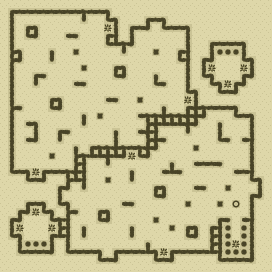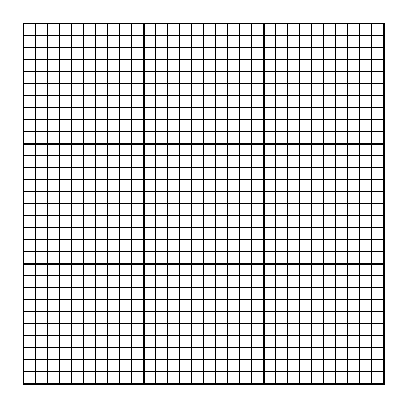### Items

•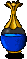4 Clear Blue Potions (18, 2) - (27, 15) - (7, 16) - (7, 23)
•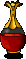Bubbling Red Potion (2, 15)

### Monsters

•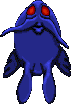14 Greater Nightmares (Random rate: 1) (18, 2) - (12, 8) - (3, 10) - (18, 11) - (10, 14) - (27, 14) - (2, 15) - (10, 16) - (28, 16) - (7, 17) - (22, 19) - (7, 23) - (8, 26) - (28, 28)
•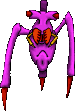46 Venemous Skitters (1, 1) - (4, 2) - (17, 3) - (18, 3) - (18, 3) - (21, 4) - (7, 5) - (6, 6) - (7, 6) - (8, 6) - (7, 7) - (4, 9) - (18, 12) - (1, 13) - (1, 14) - (16, 14) - (24, 14) - (29, 14) - (1, 15) - (8, 15) - (27, 15) - (27, 15) - (28, 15) - (1, 16) - (7, 16) - (8, 16) - (9, 16) - (9, 16) - (12, 16) - (28, 16) - (1, 17) - (1, 17) - (19, 17) - (21, 17) - (2, 18) - (24, 21) - (8, 22) - (20, 22) - (20, 22) - (21, 22) - (21, 22) - (21, 22) - (16, 24) - (24, 28) - (8, 29) - (16, 29)
•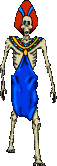Ghasts (Random rate: 6)

### Locations

• Save rune(28, 24)

### Teleporters

• (12, 2) Teleporter to (29, 7)
• (25, 7) Teleporter to (22, 11)
• (29, 7) Teleporter to (12, 2)
• (27, 9) Teleporter to (3, 25)
• (22, 11) Teleporter to (25, 7)
• (15, 18) Teleporter to (1, 27)
• (3, 25) Teleporter to (27, 9)
• (1, 27) Teleporter to (15, 18)
• (5, 27) Teleporter to (19, 30)
• (19, 30) Teleporter to (5, 27)